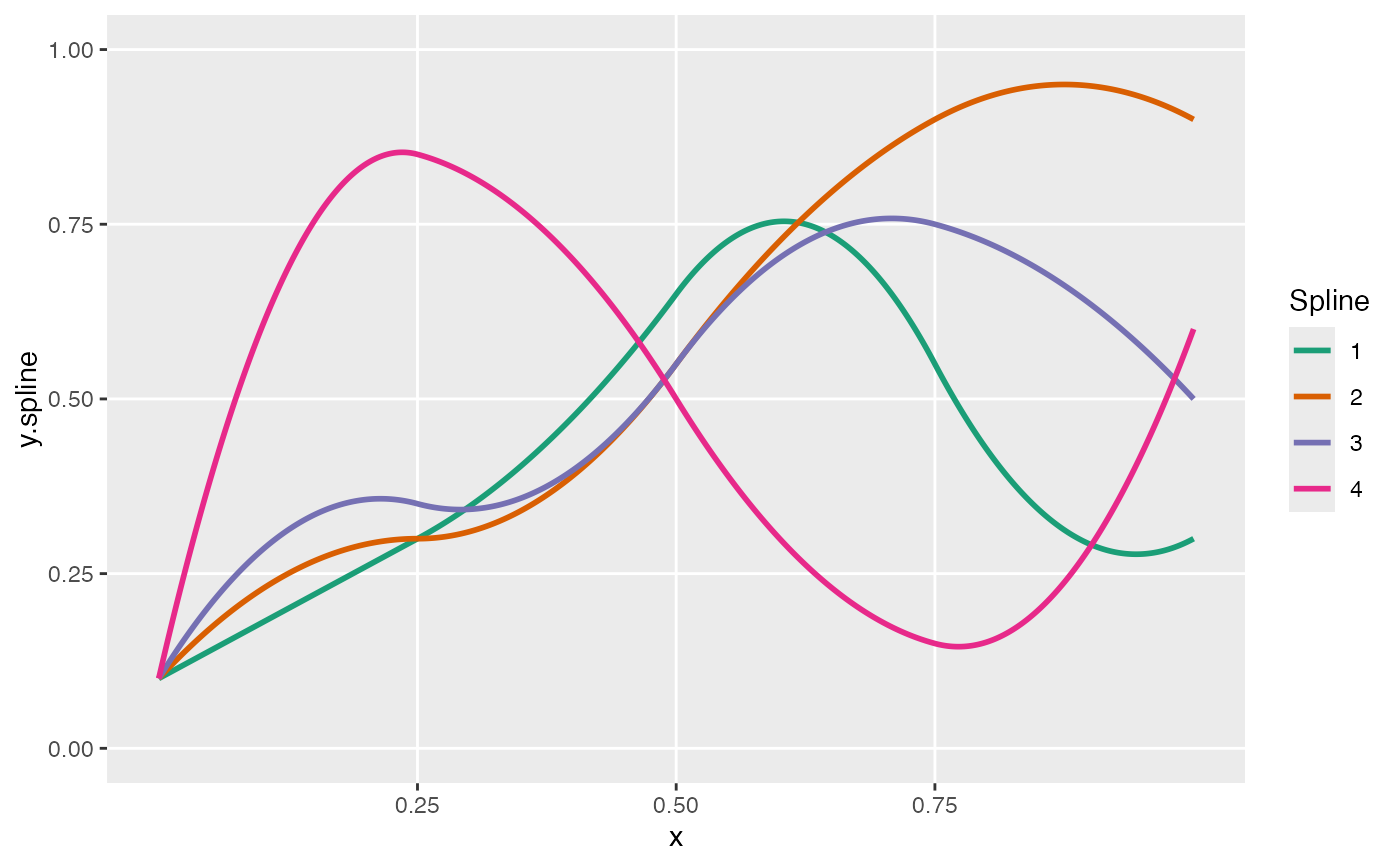Plot spline curves

viewSplines(knots, degree, theta)

## Arguments

knots

A vector of values between 0 and 1, specifying cut-points for splines

degree

Integer specifying degree of curvature.

theta

A vector or matrix of values between 0 and 1. Each column of the matrix represents the weights/coefficients that will be applied to the basis functions determined by the knots and degree. Each column of theta represents a separate spline curve.

## Value

A ggplot object that contains a plot of the spline curves. The number of spline curves in the plot will equal the number of columns in the matrix (or it will equal 1 if theta is a vector).

## Examples

knots <- c(0.25, 0.5, 0.75)
theta1 <- c(0.1, 0.8, 0.4, 0.9, 0.2, 1.0)

viewSplines(knots, degree = 2, theta1)theta2 <- matrix(c(
0.1, 0.2, 0.4, 0.9, 0.2, 0.3,
0.1, 0.3, 0.3, 0.8, 1.0, 0.9,
0.1, 0.4, 0.3, 0.8, 0.7, 0.5,
0.1, 0.9, 0.8, 0.2, 0.1, 0.6
),
ncol = 4
)

viewSplines(knots, degree = 2, theta2)